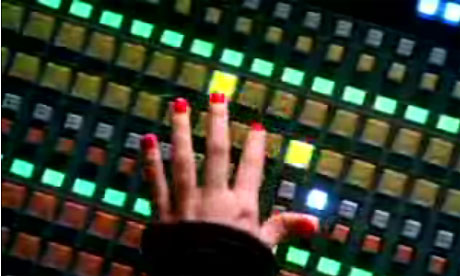# Don't push my buttons!Jenny is the operator of a large garbage disposal machine. It is a fairly simple machine and constitutes of only three buttons: One $\color{#20A900} {\text{green}}$ button, one $\color{#3D99F6} {\text{blue}}$ button and one $\color{#D61F06} {\text{red}}$ button.

Given that initially $n$ items are put in the machine, it will only destroy garbage in the three following ways:

• The $\color{#20A900} {\text{green}}$ button, if pressed destroys $\frac{1}{2}$ of the items,leaving $\frac{n}{2}$ of the items, but it only works if the number of items $n$ is divisible by $2.$
• The $\color{#3D99F6} {\text{blue}}$ button,if pressed destroys $\frac{2}{3}$ of the items,leaving $\frac{n}{3}$ items, but it only works if the number of items $n$ is divisible by $3.$
• The $\color{#D61F06} {\text{red}}$ button always works and destroys only $1$ item leaving $n-1$ if pressed.

Since Jenny is a lazy operator she wants to minimize the number of times she presses the buttons? What is the minimum number of times Jenny has to press the buttons in order to completely destroy $466559$ items?

Examples
As explicit examples for $n=10$ items Jenny would have to do $4$ button presses ($\color{#D61F06} {\text{red}}$,$\color{#3D99F6} {\text{blue}}$, $\color{#3D99F6} {\text{blue}}$,$\color{#D61F06} {\text{red}}$) to minimize the number of presses as shown below.
$10-1=9 \longrightarrow$ $9\diagup 3=3 \longrightarrow$$3\diagup3=1 \longrightarrow$$1-1=0$

For $n=6$ items the optimal solution is $3$ presses ($\color{#3D99F6} {\text{blue}}$, $\color{#20A900} {\text{green}}$,$\color{#D61F06} {\text{red}}$) or ($\color{#3D99F6} {\text{blue}}$,$\color{#D61F06} {\text{red}}$,$\color{#D61F06} {\text{red}}$)
$6\diagup 3 \longrightarrow 2 \diagup 2 \longrightarrow 1 - 1=0$ or $6\diagup 3 \longrightarrow 2 - 1 \longrightarrow 1 - 1=0$

×